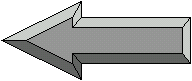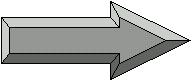gcsescience.com                                       57                                       gcsescience.com

Electricity

Revision Questions for Electricity

The best way to remember the information in this chapter
is to get a pen and paper and write down your answers
before clicking on the Answer link which will take you to the correct page.

You may have to read through some of the page before you find the answer.
If the answer you have written is not right, change it to the
correct answer by copying down the information from the correct page.

 Electricity - Series Circuits 44 Draw a Circuit with three Resistors connected in Series. Answer 45 How does Current change for Components in a Series Circuit? Answer 46 How does Voltage change for Components in a Series Circuit? Answer 47 How do you calculate the Total Resistance for Components in a Series Circuit? Answer 48 How do you calculate the Voltage for Components in a Series Circuit? Answer 49 When More Lamps are connected in Series, do they get Dimmer or Brighter? Answer 50 If one Lamp Breaks in a Series Circuit, will the others stay On or go Out? Answer

 Electricity - Parallel Circuits 51 Draw a Circuit with three Resistors connected in Parallel. Answer 52 How does Current change for Components in a Parallel Circuit? Answer 53 How do you calculate the Current in each Branch of a Parallel Circuit? Answer 54 How do you calculate the Total Current for Components in a Parallel Circuit? Answer 55 How does Voltage change for Components in a Parallel Circuit? Answer 56 How do you calculate the Total Resistance for Components in a Parallel Circuit? Answer 57 Draw a Circuit with three Lamps connected in Parallel. Answer 58 How can you Switch each Lamp On or Off without affecting the other Lamps? Answer 59 What is the Circuit Symbol for an Open Switch? Answer 60 What is the Circuit Symbol for a Closed Switch? AnswerLinks      Electricity      Mains      Calculations